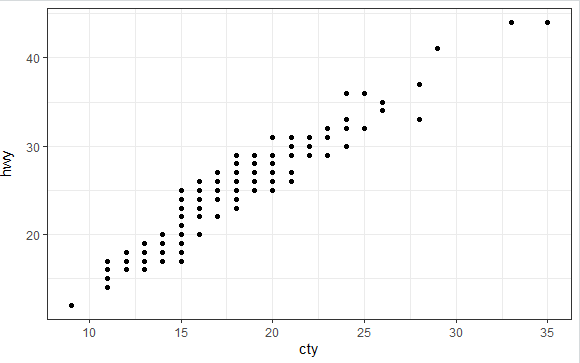2 views

The y-axis title appears too close to the axis text.

ggplot(mpg, aes(cty, hwy)) + geom_point()I have tried changing the value of many parameters with the theme() but none seems to help.

by

You can use the margin argument of the element.text function to change the distance between the axis text and axis title.i.e.,

ggplot(mpg, aes(cty, hwy)) + geom_point()+

theme(axis.title.y = element_text(margin = margin(t = 0, r = 10, b = 0, l = 0)))

Set the values of the margin on top(t), right(r), bottom(b), and left(l) side of the element.

Output: